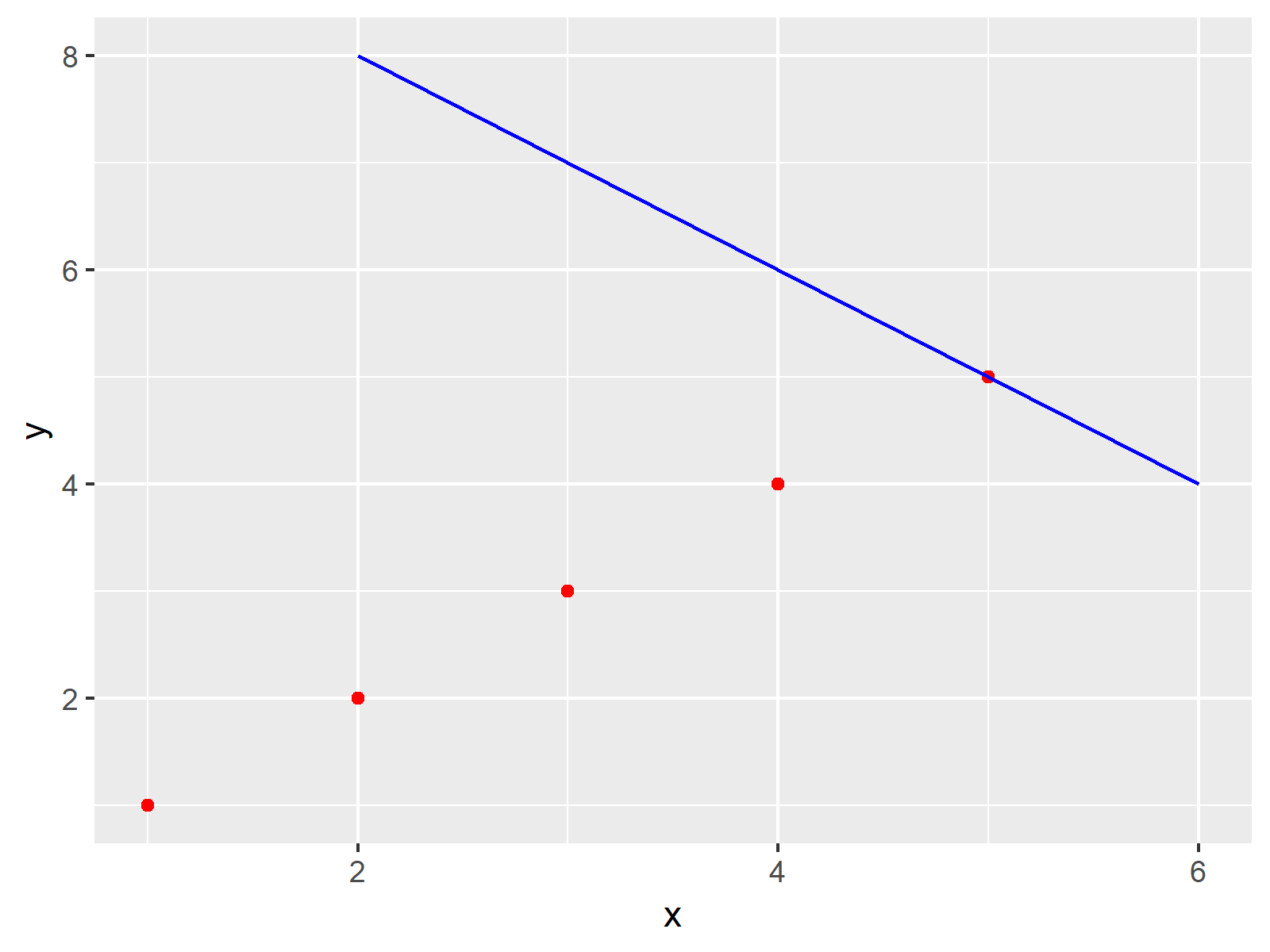# Combine Two ggplot2 Plots from Different Data Frames in R (Example)

In this article you’ll learn how to draw a ggplot2 plot based on several different data sources in the R programming language.

Let’s do this…

## Example Data, Add-On Packages & Default Plot

Consider the following example data:

```data1 <- data.frame(x = 1:5, # Create first data frame y = 1:5) data1 # Print first data frame # x y # 1 1 1 # 2 2 2 # 3 3 3 # 4 4 4 # 5 5 5 data2 <- data.frame(x = 2:6, # Create second data frame y = 8:4) data2 # Print second data frame # x y # 1 2 8 # 2 3 7 # 3 4 6 # 4 5 5 # 5 6 4```

The previous RStudio console output shows the structure of our example data sets – Both data frames contains two numeric columns with the variable names x and y.

If we want to use the functions of the ggplot2 package, we also have to install and load ggplot2:

```install.packages("ggplot2") # Install ggplot2 package library("ggplot2") # Load ggplot2 package```

Now, we can move on to the example…

## Example: Drawing ggplot2 Plot Based on Two Different Data Frames

This Section shows how to use the ggplot2 package to draw a plot based on two different data sets.

For this, we have to set the data argument within the ggplot function to NULL. Then, we are specifying two geoms (i.e. geom_point and geom_line) and define the data set we want to use within each of those geoms.

```ggp <- ggplot(NULL, aes(x, y)) + # Draw ggplot2 plot based on two data frames geom_point(data = data1, col = "red") + geom_line(data = data2, col = "blue") ggp # Draw plot```Figure 1 visualizes the output of the previous R code – A ggplot2 graph created based on multiple different data matrices.

## Video, Further Resources & Summary

Furthermore, I can recommend to have a look at some of the other tutorials of this homepage:

Summary: In this article, I explained how to create a ggplot2 graph with two different data sets in the R programming language. Let me know in the comments section, in case you have additional questions.